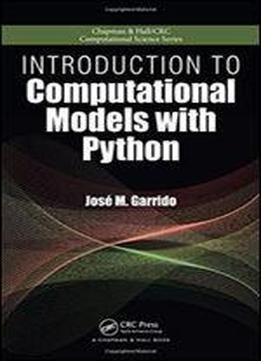# Introduction To Computational Models With Python by Jose M. Garrido / 2017 / English / PDF

Introduction to Computational Models with Python explains how to implement computational models using the flexible and easy-to-use Python programming language. The book uses the Python programming language interpreter and several packages from the huge Python Library that improve the performance of numerical computing, such as the Numpy and Scipy modules. The Python source code and data files are available on the authors website. The books five sections present: An overview of problem solving and simple Python programs, introducing the basic models and techniques for designing and implementing problem solutions, independent of software and hardware tools Programming principles with the Python programming language, covering basic programming concepts, data definitions, programming structures with flowcharts and pseudo-code, solving problems, and algorithms Python lists, arrays, basic data structures, object orientation, linked lists, recursion, and running programs under Linux Implementation of computational models with Python using Numpy, with examples and case studies The modeling of linear optimization problems, from problem formulation to implementation of computational models This book introduces the principles of computational modeling as well as the approaches of multi- and interdisciplinary computing to beginners in the field. It provides the foundation for more advanced studies in scientific computing, including parallel computing using MPI, grid computing, and other methods and techniques used in high-performance computing.

views: 409